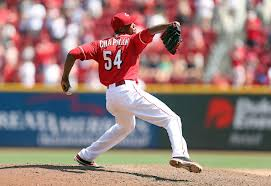# Pitching Velocity and The Physics Behind It##### Vf=Vi + At
###### Final Velocity=Initial Velocity + Acceleration (Time)

Final velocity is a product of initial velocity plus the acceleration force over a period of time. The longer you apply an acceleration force to the ball, the greater the final velocity. This correlates to stride length and amount of time the hand is in contact with the ball. We will show you how to maximize your stride length and use your arm correctly to accelerate the ball efficiently.

###### Force=Mass x Acceleration

In baseball, the mass is constant and the acceleration force occurs in a linear and angular direction. Maximizing your acceleration is a product of angular acceleration and linear acceleration. Understanding how to accomplish this requires knowledge of how the length of a lever arm affects your ability to create both.   This is the foundation of our phase specific throwing program. You will see how correct placement of the ball during the transitions from phase to phase will affect your ability to maximize angular and linear velocity.

##### Angular Velocity runs tangential to an arc.

Maximizing angular velocity requires that the distance of your radius on that arc be shortened at exactly the right time during the throwing motion. This is the equivalent of the analogy we used before of cracking a whip. Accelerating a ball along an arc and maximizing the linear displacement of the ball allows you to accelerate the ball for the greatest amount of time. This will result in a maximized final velocity. This is the goal of our program.

Eccentric and Concentric muscle loading, stored potential energy converted to kinetic energy, a perfectly timed Kinematic sequence, maximized linear and angular acceleration, and a clinical understanding of how our structural anatomy needs to be used is what you find at the OAI and nowhere else.BaseballKeys to Velocitykinematic sequencephysicsPitching